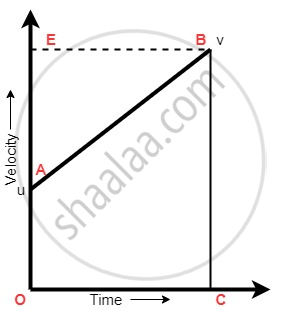Share

# Equations of Motion by Graphical Method - Equation for Position-velocity Relation

#### notes

Equation of position – Velocity Relation (3rd equation of motion)Area under slope = Displacement = Area of OABC

"Area of trapezium OABC" = 1/2 xx ("BC + OA") xx "OC"

"S" = 1/2 xx ("v + u") xx "t"

By 1st eq of motion   v = u + at

"S"= 1/2 xx ("v + u") xx ("v – u"/"a") ["v - u"/"a" = "t"]

"S" = "(v + u)" "(v – u)"/ "2a" "(a + b) (a – b)" = "a"^2 – "b"^2

"2as" = "v - u"

"v"^2 ="u"^2- 2"as"

S